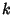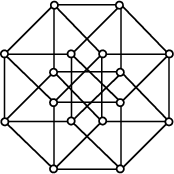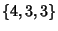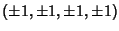## HypercubeThe generalization of a 3-Cube to-D, also called a Measure Polytope. It is a regular Polytope with mutually Perpendicular sides, and is therefore an Orthotope. It is denotedand has Schläfli Symbol. The number of-cubes contained in an-cube can be found from the Coefficients of.The 1-hypercube is a Line Segment, the 2-hypercube is the Square, and the 3-hypercube is the Cube. The hypercube in, called a Tesseract, has the Schläfli Symboland Vertices. The above figures show two visualizations of the Tesseract. The figure on the left is a projection of the Tesseract in 3-space (Gardner 1977), and the figure on the right is the Graph of the Tesseract symmetrically projected into the Plane (Coxeter 1973). A Tesseract has 16 Vertices, 32 Edges, 24 Squares, and eight Cubes.

References

Coxeter, H. S. M. Regular Polytopes, 3rd ed. New York: Dover, p. 123, 1973.

Gardner, M. Hypercubes.'' Ch. 4 in Mathematical Carnival: A New Round-Up of Tantalizers and Puzzles from Scientific American. New York: Vintage Books, 1977.

Geometry Center. The Tesseract (or Hypercube).'' http://www.geom.umn.edu/docs/outreach/4-cube/.

Pappas, T. How Many Dimensions are There?'' The Joy of Mathematics. San Carlos, CA: Wide World Publ./Tetra, pp. 204-205, 1989.